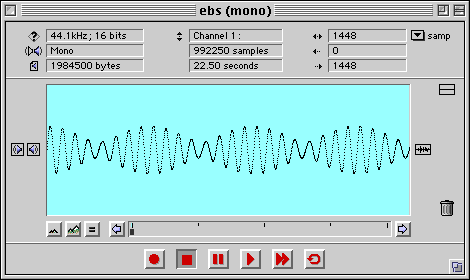The Physics
Hypertextbook
Opus in profectus

# Beats

## Practice

### practice problem 1

A 32.83 ms fragment of the EBS alert tone is shown in the oscilloscope display below. (The full tone lasts 22.5 seconds.) The beat structure is plainly visible at this scale. There are approximately 3.5 cycles of the beat and 29.75 cycles of the carrier visible in the display window. Determine the frequencies of the two tones used to produce the alert.#### solution

Start by determining the beat and carrier frquencies from the information given in the description.

 fbeat = 3.5 / 0.03283 s = 107 Hz fcarrier = 29.75 / 0.03283 s = 906 Hz

Then work backward to determine the two source frequencies. The beat is the difference in two frequencies and the carrier is their mean.

 fbeat = f1 − f2 = 107 Hz fcarrier = f1 + f2 = 906 Hz 2

A little bit of algebra on the carrier equation gives us two equations that can be added. Watch one of the unknowns vanish.

 f1 − f2 = 0107 Hz f1 + f2 = 1812 Hz 2f1 = 1919 Hz

And we're done.

 f1 = 960 Hz
 f2 = 853 Hz

These are indeed the values specified by US Federal law.

### practice problem 2

Write something else.

### practice problem 3

Write something different.